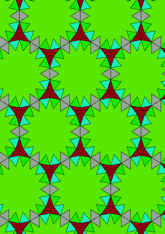# Derived from Figure 2.5.4 (p) by adding trianglesdata146/F417D

## Geometry

• The symmetry group of the tiling is *632 (p6m).
• All the internal angles of the constituent polygons are a multiple of 20°.
• Contains three equilateral triangles.
• Contains one regular three-pointed star polygon with vertex angle of 40°.
• Contains one regular 18-pointed star polygon with vertex angle of 40°.
• The tiling does not satisfy the two-colour condition.
• The tiling is edge-to-edge.
• As drawn, contains about 383 polygons.

## References

Publications referenced:
1. of B A Wichmann. The World of Patterns, World Scientific, 2001. ISBN 981024619. [wich2] {Only new patterns ascribed to this.}

v53